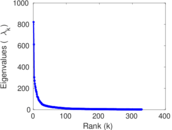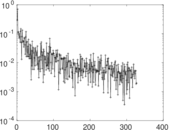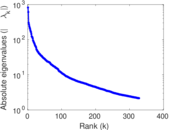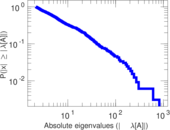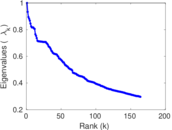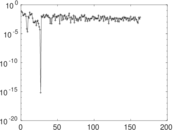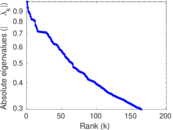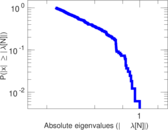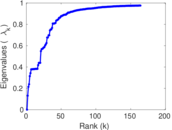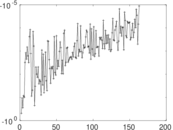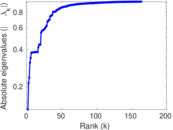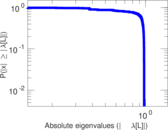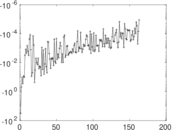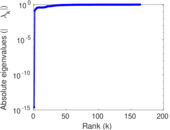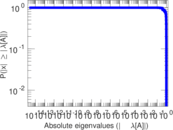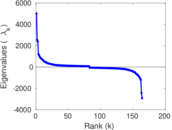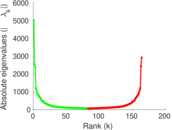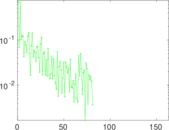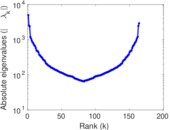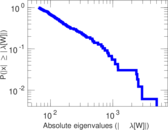# Wikipedia edits (fy)

This is the bipartite edit network of the Western Frisian Wikipedia. It contains users and pages from the Western Frisian Wikipedia, connected by edit events. Each edge represents an edit. The dataset includes the timestamp of each edit.

 Code `fy` Internal name `edit-fywiki` Name Wikipedia edits (fy) Data source http://dumps.wikimedia.org/ AvailabilityDataset is available for download Consistency checkDataset passed all tests Category Authorship network Dataset timestamp 2017-10-20 Node meaning User, article Edge meaning Edit Network formatBipartite, undirected Edge typeUnweighted, multiple edges Temporal dataEdges are annotated with timestamps

## Statistics

 Size n = 4,551 Left size n1 = 1,041 Right size n2 = 3,510 Volume m = 161,936 Unique edge count m̿ = 55,821 Wedge count s = 25,945,514 Claw count z = 10,483,037,798 Cross count x = 3,847,537,848,418 Square count q = 182,347,655 4-Tour count T4 = 1,562,731,962 Maximum degree dmax = 12,382 Maximum left degree d1max = 12,382 Maximum right degree d2max = 1,983 Average degree d = 71.165 0 Average left degree d1 = 155.558 Average right degree d2 = 46.135 6 Fill p = 0.015 277 1 Average edge multiplicity m̃ = 2.900 99 Size of LCC N = 4,502 Diameter δ = 9 50-Percentile effective diameter δ0.5 = 2.303 59 90-Percentile effective diameter δ0.9 = 3.731 65 Median distance δM = 3 Mean distance δm = 2.907 85 Gini coefficient G = 0.788 820 Balanced inequality ratio P = 0.206 270 Left balanced inequality ratio P1 = 0.057 238 7 Right balanced inequality ratio P2 = 0.261 591 Relative edge distribution entropy Her = 0.807 306 Power law exponent γ = 1.556 63 Tail power law exponent γt = 1.431 00 Tail power law exponent with p γ3 = 1.431 00 p-value p = 0.000 00 Left tail power law exponent with p γ3,1 = 1.531 00 Left p-value p1 = 0.000 00 Right tail power law exponent with p γ3,2 = 7.441 00 Right p-value p2 = 0.043 000 0 Degree assortativity ρ = −0.181 380 Degree assortativity p-value pρ = 0.000 00 Spectral norm α = 821.519 Algebraic connectivity a = 0.131 173 Spectral separation |λ1[A] / λ2[A]| = 1.343 30 Controllability C = 2,940 Relative controllability Cr = 0.651 019

## Plots

### Fruchterman–Reingold graph drawing### Degree distribution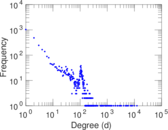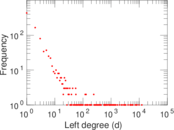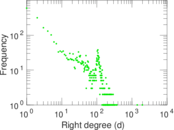### Cumulative degree distribution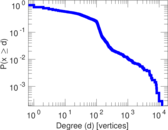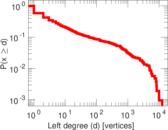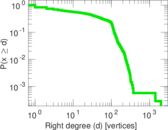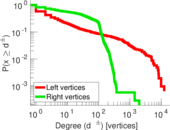### Lorenz curve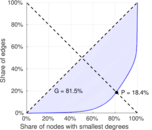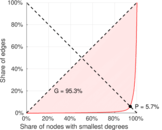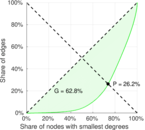### Spectral distribution of the adjacency matrix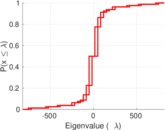### Spectral distribution of the normalized adjacency matrix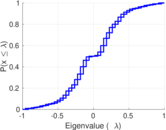### Spectral distribution of the Laplacian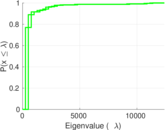### Spectral graph drawing based on the adjacency matrix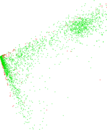### Spectral graph drawing based on the Laplacian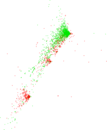### Spectral graph drawing based on the normalized adjacency matrix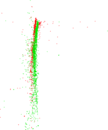### Degree assortativity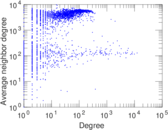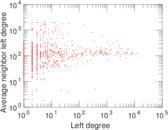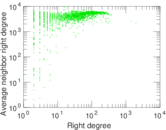### Zipf plot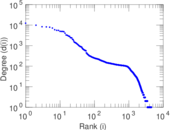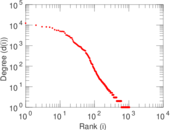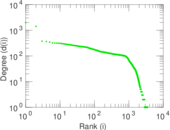### Hop distribution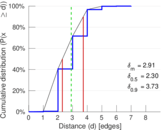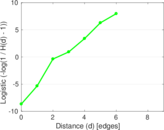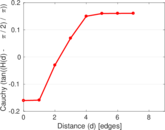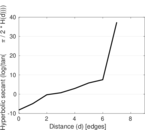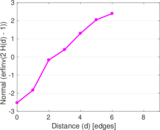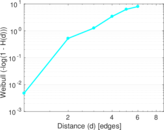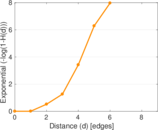### Double Laplacian graph drawing### Delaunay graph drawing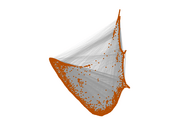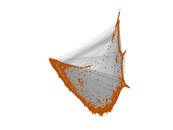### Edge weight/multiplicity distribution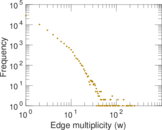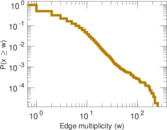### Temporal distribution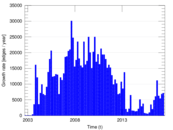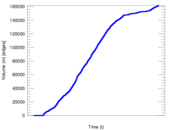### Temporal hop distribution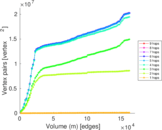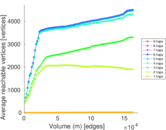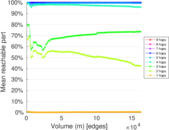### Diameter/density evolution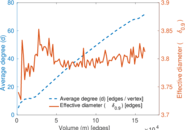### Matrix decompositions plots